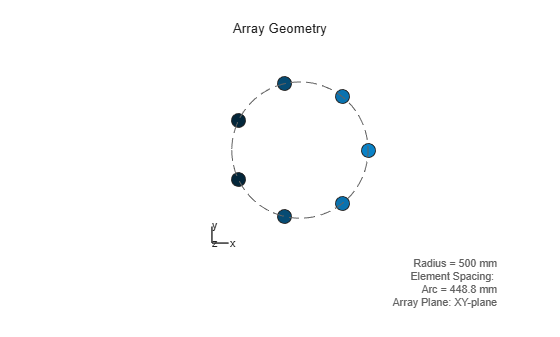# getTaper

System object: phased.UCA
Package: phased

Array element tapers

## Syntax

``` WTS = getTaper(H) ```

## Description

`WTS = getTaper(H)` returns the tapers, `WTS`, applied to each element of the phased uniform circular array (UCA), `H`. Tapers are often referred to as weights.

## Input Arguments

expand all

Uniform circular array, specified as a `phased.ULA` System object.

Example: `H = phased.UCA();`

## Output Arguments

expand all

Array element tapers, returned as an N-by-1 complex-valued vector, where N is the number of elements in the array.

## Examples

expand all

Construct a 7-element UCA array of isotropic antenna elements with a Taylor window taper. Design the array to have a radius of 0.5 meters. Then, draw the array showing the element taper shading.

```Nelem = 7; R = 0.5; taper = taylorwin(Nelem); sArray = phased.UCA(Nelem,R,'Taper',taper.'); w = getTaper(sArray)```
```w = 7×1 0.4520 0.9009 1.3680 1.5581 1.3680 0.9009 0.4520 ```
`viewArray(sArray,'ShowTaper',true);`Both the output and figure above shows that the taper magnitudes are largest near the middle element.

Introduced in R2015a